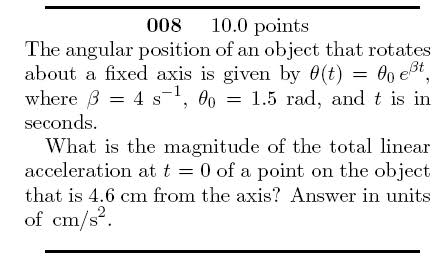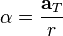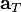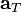# Angular Acceleration Problem

## Homework Statement## The Attempt at a Solution

I thought I could take the second derivative and get the angular acceleration from which I could usewhere ω is the angular velocity,is the linear tangential acceleration, and r is the radius of curvature. But for some reason, it didn't give me the right answer, anyone know where I went wrong?

alphysicist
Homework Helper
Hi Garen,

## Homework Statement## The Attempt at a Solution

I thought I could take the second derivative and get the angular acceleration from which I could usewhere ω is the angular velocity,is the linear tangential acceleration, and r is the radius of curvature. But for some reason, it didn't give me the right answer, anyone know where I went wrong?

Are you saying that you put in the tangential acceleration as your answer? If so, remember that they are asking for the total linear acceleration, and there is more to the total acceleration than just the tangential part.

aaaah thank you so much. i was totally stalking this post and you replied to it in a MOMENT OF PARADISE!!!!!

Hi Garen,

Are you saying that you put in the tangential acceleration as your answer? If so, remember that they are asking for the total linear acceleration, and there is more to the total acceleration than just the tangential part.

Oh, I thought that total linear acceleration was only the tangential acceleration...Would I have to include centripetal acceleration? If so, how?

alphysicist
Homework Helper
Oh, I thought that total linear acceleration was only the tangential acceleration...Would I have to include centripetal acceleration? If so, how?

What is the formula for centripetal acceleration? (And remember that you have already found the angular velocity!)

Once you have found both components (the tangential and centripetal), the total is just the vector sum.

What is the formula for centripetal acceleration? (And remember that you have already found the angular velocity!)

Once you have found both components (the tangential and centripetal), the total is just the vector sum.

I got it! Thanks a lot for your help.

alphysicist
Homework Helper
I got it! Thanks a lot for your help.